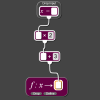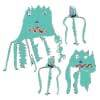# Resources tagged with: Place value

Filter by: Content type:
Age range:
Challenge level:

### There are 64 results

Broad Topics > The Number System and Place Value > Place value### Enriching Experience

##### Age 14 to 16 Challenge Level:

Find the five distinct digits N, R, I, C and H in the following nomogram### Football Sum

##### Age 11 to 14 Challenge Level:

Find the values of the nine letters in the sum: FOOT + BALL = GAME### Two and Two

##### Age 11 to 16 Challenge Level:

How many solutions can you find to this sum? Each of the different letters stands for a different number.### Think of Two Numbers

##### Age 11 to 14 Challenge Level:

Think of two whole numbers under 10, and follow the steps. I can work out both your numbers very quickly. How?### What an Odd Fact(or)

##### Age 11 to 14 Challenge Level:

Can you show that 1^99 + 2^99 + 3^99 + 4^99 + 5^99 is divisible by 5?### Eleven

##### Age 11 to 14 Challenge Level:

Replace each letter with a digit to make this addition correct.### More Mathematical Mysteries

##### Age 11 to 14 Challenge Level:

Write down a three-digit number Change the order of the digits to get a different number Find the difference between the two three digit numbers Follow the rest of the instructions then try. . . .### Quick Times

##### Age 11 to 14 Challenge Level:

32 x 38 = 30 x 40 + 2 x 8; 34 x 36 = 30 x 40 + 4 x 6; 56 x 54 = 50 x 60 + 6 x 4; 73 x 77 = 70 x 80 + 3 x 7 Verify and generalise if possible.### Cayley

##### Age 11 to 14 Challenge Level:

The letters in the following addition sum represent the digits 1 ... 9. If A=3 and D=2, what number is represented by "CAYLEY"?### Number Rules - OK

##### Age 14 to 16 Challenge Level:

Can you convince me of each of the following: If a square number is multiplied by a square number the product is ALWAYS a square number...### Really Mr. Bond

##### Age 14 to 16 Challenge Level:

115^2 = (110 x 120) + 25, that is 13225 895^2 = (890 x 900) + 25, that is 801025 Can you explain what is happening and generalise?##### Age 11 to 14 Challenge Level:

Replace the letters with numbers to make the addition work out correctly. R E A D + T H I S = P A G E### Reversals

##### Age 11 to 14 Challenge Level:

Where should you start, if you want to finish back where you started?### Chocolate Maths

##### Age 11 to 14 Challenge Level:

Pick the number of times a week that you eat chocolate. This number must be more than one but less than ten. Multiply this number by 2. Add 5 (for Sunday). Multiply by 50... Can you explain why it. . . .##### Age 11 to 14 Challenge Level:

By selecting digits for an addition grid, what targets can you make?### Never Prime

##### Age 14 to 16 Challenge Level:

If a two digit number has its digits reversed and the smaller of the two numbers is subtracted from the larger, prove the difference can never be prime.### Mini-max

##### Age 11 to 14 Challenge Level:

Consider all two digit numbers (10, 11, . . . ,99). In writing down all these numbers, which digits occur least often, and which occur most often ? What about three digit numbers, four digit numbers. . . .### Plus Minus

##### Age 14 to 16 Challenge Level:

Can you explain the surprising results Jo found when she calculated the difference between square numbers?### Three Times Seven

##### Age 11 to 14 Challenge Level:

A three digit number abc is always divisible by 7 when 2a+3b+c is divisible by 7. Why?### Always a Multiple?

##### Age 11 to 14 Challenge Level:

Think of a two digit number, reverse the digits, and add the numbers together. Something special happens...### The Number Jumbler

##### Age 7 to 14 Challenge Level:

The Number Jumbler can always work out your chosen symbol. Can you work out how?### Repeaters

##### Age 11 to 14 Challenge Level:

Choose any 3 digits and make a 6 digit number by repeating the 3 digits in the same order (e.g. 594594). Explain why whatever digits you choose the number will always be divisible by 7, 11 and 13.### Multiplication Magic

##### Age 14 to 16 Challenge Level:

Given any 3 digit number you can use the given digits and name another number which is divisible by 37 (e.g. given 628 you say 628371 is divisible by 37 because you know that 6+3 = 2+7 = 8+1 = 9). . . .### DOTS Division

##### Age 14 to 16 Challenge Level:

Take any pair of two digit numbers x=ab and y=cd where, without loss of generality, ab > cd . Form two 4 digit numbers r=abcd and s=cdab and calculate: {r^2 - s^2} /{x^2 - y^2}.### Subtraction Surprise

##### Age 7 to 14 Challenge Level:

Try out some calculations. Are you surprised by the results?### Arrange the Digits

##### Age 11 to 14 Challenge Level:

Can you arrange the digits 1,2,3,4,5,6,7,8,9 into three 3-digit numbers such that their total is close to 1500?### Digit Sum

##### Age 14 to 16 Short Challenge Level:

What is the sum of all the digits in all the integers from one to one million?### How Many Miles to Go?

##### Age 11 to 14 Challenge Level:

How many more miles must the car travel before the numbers on the milometer and the trip meter contain the same digits in the same order?### Just Repeat

##### Age 11 to 14 Challenge Level:

Think of any three-digit number. Repeat the digits. The 6-digit number that you end up with is divisible by 91. Is this a coincidence?### Big Powers

##### Age 11 to 16 Challenge Level:

Three people chose this as a favourite problem. It is the sort of problem that needs thinking time - but once the connection is made it gives access to many similar ideas.##### Age 11 to 14 Challenge Level:

What happens when you add a three digit number to its reverse?### Exploring Simple Mappings

##### Age 11 to 14 Challenge Level:

Explore the relationship between simple linear functions and their graphs.### What a Joke

##### Age 14 to 16 Challenge Level:

Each letter represents a different positive digit AHHAAH / JOKE = HA What are the values of each of the letters?##### Age 11 to 14 Challenge Level:

Visitors to Earth from the distant planet of Zub-Zorna were amazed when they found out that when the digits in this multiplication were reversed, the answer was the same! Find a way to explain. . . .### Diagonal Sums

##### Age 7 to 14 Challenge Level:

In this 100 square, look at the green square which contains the numbers 2, 3, 12 and 13. What is the sum of the numbers that are diagonally opposite each other? What do you notice?### Double Digit

##### Age 11 to 14 Challenge Level:### Composite Notions

##### Age 14 to 16 Challenge Level:

A composite number is one that is neither prime nor 1. Show that 10201 is composite in any base.### 2-digit Square

##### Age 14 to 16 Challenge Level:

A 2-Digit number is squared. When this 2-digit number is reversed and squared, the difference between the squares is also a square. What is the 2-digit number?### Novemberish

##### Age 14 to 16 Challenge Level:

a) A four digit number (in base 10) aabb is a perfect square. Discuss ways of systematically finding this number. (b) Prove that 11^{10}-1 is divisible by 100.### Legs Eleven

##### Age 11 to 14 Challenge Level:

Take any four digit number. Move the first digit to the end and move the rest along. Now add your two numbers. Did you get a multiple of 11?### Back to the Planet of Vuvv

##### Age 11 to 14 Challenge Level:

There are two forms of counting on Vuvv - Zios count in base 3 and Zepts count in base 7. One day four of these creatures, two Zios and two Zepts, sat on the summit of a hill to count the legs of. . . .### Reach 100

##### Age 7 to 14 Challenge Level:

Choose four different digits from 1-9 and put one in each box so that the resulting four two-digit numbers add to a total of 100.### Back to Basics

##### Age 14 to 16 Challenge Level:

Find b where 3723(base 10) = 123(base b).### Seven Up

##### Age 11 to 14 Challenge Level:

The number 27 is special because it is three times the sum of its digits 27 = 3 (2 + 7). Find some two digit numbers that are SEVEN times the sum of their digits (seven-up numbers)?### Tis Unique

##### Age 11 to 14 Challenge Level:

This addition sum uses all ten digits 0, 1, 2...9 exactly once. Find the sum and show that the one you give is the only possibility.### Latin Numbers

##### Age 14 to 16 Challenge Level:

Can you create a Latin Square from multiples of a six digit number?### Basically

##### Age 11 to 14 Challenge Level:

The number 3723(in base 10) is written as 123 in another base. What is that base?### Nice or Nasty for Two

##### Age 7 to 14 Challenge Level:

Some Games That May Be Nice or Nasty for an adult and child. Use your knowledge of place value to beat your opponent.### Writ Large

##### Age 11 to 14 Challenge Level:

Suppose you had to begin the never ending task of writing out the natural numbers: 1, 2, 3, 4, 5.... and so on. What would be the 1000th digit you would write down.### Lesser Digits

##### Age 11 to 14 Challenge Level:

How many positive integers less than or equal to 4000 can be written down without using the digits 7, 8 or 9?